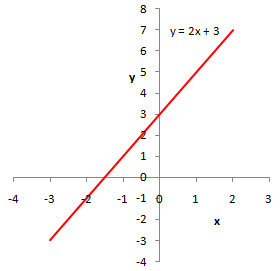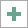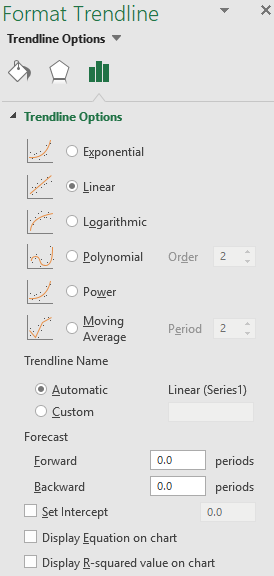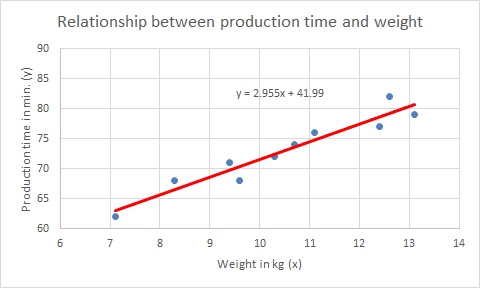# 17 Measurements

• Explanation of linear relations.
• Add a linear trendline to a scatter chart.
• Worksheet functions SLOPE and INTERCEPT.

Researchers often want to have an answer to the question of whether the value of a variable depends on the value of another variable. To find out this, they carry out measurements. When performing these measurements, the value of one variable is changed (the independent variable) and the value of another variable (the dependent variable) is measured.

These measurements provide a series of readings that can be plotted in a chart. The course of the chart gives often a first impression of whether there is a relationship between the two variables and, if so, what kind of relationship: linear, exponential, logarithmic, …

A relationship between variables is represented by a mathematical function and indicated by an equation. When the relationship is linear, the chart is a straight line.

When plotting the measurement results, there will mostly be no line that fits all points. Using statistical methods, applications like Excel can determine the best fitting line. Such a line is called a regression line. The calculation method for finding the best line is known as the least-squares method. The backgrounds of this method and the statistical techniques are not treated. If you want to know more you have to study the literature.

## 17.1 Linear relationship

If there is a linear relationship between two variables $$x$$ and $$y$$ then the relation between these two variables can be represented by the equation

$$y = ax + b$$

• $$y$$ is the dependent variable
• $$x$$ is the independent variable
• $$a$$ is the slope, a constant
• $$b$$ is the intercept, a constantFigure 17.1: The graph of y=2x+3 is a straight line.

The slope of the line, a, is 2, and the intercept (intersection with the y-axis),b, is 3.

With measurement results you can use Excel to

• draw a chart of the points.
• draw a trend line (the best fitting line).
• determine the equation of the trendline.
• determine the slope and intercept with Excel functions.

## 17.2 Task: Linear trend line

Explanation of how to add a linear trend line.

In a timber factory, a large number of similar items are manufactured, which mainly show differences in their dimensions and weight. To investigate whether the production time of these items depends on their weight, several measurements are performed. A linear relationship is assumed.

File: Prodtime_Weight.xlsx

1. Open the practice file.

2. Select the area with the measurements, A2:B11.

3. Choose tab Insert > Recommended Charts (group Charts) > Scatter > OK.

4. Add a linear trend line.

You can add a trendline in the following ways.

• Select the points in the chart, then right click and choose Add Trendline.
• Select the chart area and Click the buttonnext to the chart and choose Trendline > Linear.
1. Select trend line, Right click > Format Trendline.

2. In the Format Trendline task pane, select [Display equation on chart] {.uicontrol}.Figure 17.2: Trendline options.

1. Make the following adjustments (see the example in figure 17.3):

• Add chart title and axis titles.
• Adjust the scaling of the axes.
• No decimals in the numbers along the axes.
• Make the trendline a solid red line.
• Drag the equation to a more visible place.Figure 17.3: Scatter diagram of the measurements with a linear trendline.

From the equation of the trend line you can now determine the relationship between the two variables:

$$\text{Productiontime} = 2,955*\text{Weight} + 41,99$$

The slope of the trendline and the interception with the y-axis can be determined with the functions SLOPE and INTERCEPT from the category Statistical.

1. Select an empty cell on the worksheet and insert function SLOPE. Specify here

• Known_ys: B2:B11
• Known_xs: A2:A11
2. Determine the same way in another cell the value of INTERCEPT.

The value of the slope is 2.95503212 and the value of intersection is 41.99036403. This provides the data for the trendline equation.

## 17.3 Exercises

### meas001 - Height and Weight

The height and weight of some school children are listed in the following figure.Results height and weight

Imagine a linear relationship and determine the equation where the weight is a function from the height.

File: Meas001.xlsx

Weight = 1.65*Height - 153.3

### meas002 - Study time and exam grade

The study time and marks of some students is listed in the following figure.Results study time and marks

Imagine a linear relationship and determine the equation where the mark is a function from the study time.

File: Meas002.xlsx

Mark = 0.2038*Study time + 3.3637

### meas003 - Shrinkage and temperature

A manufacturer of synthetic fibers examines whether the shrinking of the fibers is related to the temperature at which they are washed. There are 8 experiments in which the fibers are washed at different temperatures. The measured shrinkage is listed below as a percentage of the original length.Results shrink percentage and temperature of washing

1. Imagine a linear relationship and determine the equation where the shrink percentage is a function from the temperature.
2. Predict the shrinkage at a temperature of 65oC.

File: Meas003.xlsx

Shrink percentage= 0.0796*Temp - 3.546
At a temperature of 65°C the predicted shrinkage percentage is 1.6%.

### meas004 - Resistance and temperature

The resistance of a metal block depends on the temperature among other things. In the following figure, you see the resistance at some temperatures.Resultaten weerstand en temperatuur

1. Imagine a linear relationship and determine the equation where the resistance is a function from the temperature.
2. Predict the resistance at a temperature of 400oC.

File: Meas004.xlsx

Resistance = 0.0786*Temp + 21.214
At a temperature of 400°C the predicted resistance is 52.6 Ohm.